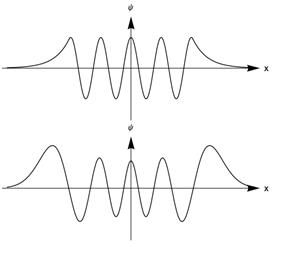## Homework for Waves

Below are two eigenfunctions of different Hamiltonian operators. Consider the Hamiltonian operators corresponding to the 5 potential wells drawn below the functions. For each function, decide whether or not it could be the eigenfunction of each the 5 Hamiltonian operators and say why. Eigenfunctions:Potential energy functions:

The x-axes of the potential functions do not correspond to those of the wave functions. The potentials correspond to, from left to right, the infinite square well (ISW), the finite square well (FSW), the quadratic or harmonic oscillator potential (HO), and a 1-sided linear potential well (1LW), and a 2-sided linear well (2LW).

##### Views

New Users

Curriculum

Pedagogy

Institutional Change

Publications

##### Toolbox